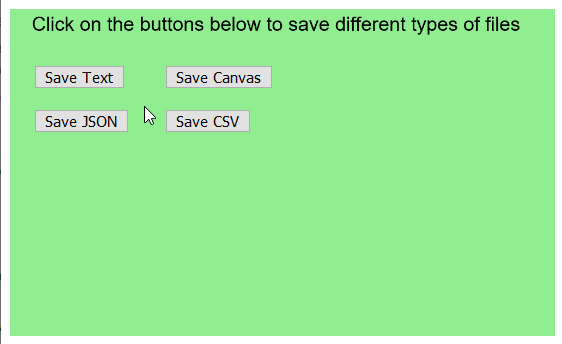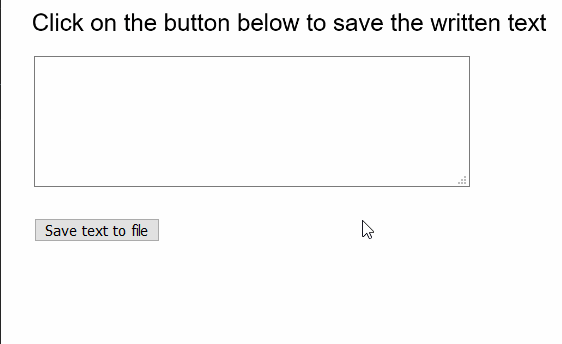Related Articles

# p5.js | save() Function

• Last Updated : 23 Aug, 2021

The save() function in p5.js is used to save to the file system by prompting a download to the computer. This function can be used to save text, images, JSON, CSV, wav, or HTML files. The default option is to save the current canvas as an image.
The first parameter of the function can be specified various values depending on the file to save. Examples include a pointer to the canvas element, an array of Strings, a JSON object, or an array, a p5.Table element for tables, a p5.Image element for images or a p5.SoundFile element for sounds.
Note: It is not recommended to call this function inside the draw() loop, as it will prompt a new save dialog every draw call.

Syntax:

`save( [objectOrFilename], [filename], [options] )`

Parameters: This function accepts three parameter as mentioned above and described below.

• objectOrFilename: This is an Object or String that is used to denote the object to save or the filename (if saving the canvas). If an Object is provided, it will save the file depending upon the object and filename. It is an optional parameter.
• filename: It specifies the String that is used as the filename of the saved file. It is an optional parameter.
• options: It is a Boolean value or String which provides additional options for the file to be saved. Incase of JSON files, a value of ‘true’ would save the JSON optimized for filesize, instead of readability. It is an optional parameter.

The examples below illustrate the save() function in p5.js:
Example 1:

## javascript

 `function` `setup() {``  ``createCanvas(500, 300);``  ``textSize(18);` `  ``background(``"lightgreen"``);``  ``text(``"Click on the buttons below to save different types of files"``, 20, 20);` `  ``// Create a button for saving text``  ``saveTextBtn = createButton(``"Save Text"``);``  ``saveTextBtn.position(30, 60);``  ``saveTextBtn.mousePressed(saveAsText);` `  ``// Create a button for saving canvas image``  ``saveImageBtn = createButton(``"Save Canvas"``);``  ``saveImageBtn.position(150, 60);``  ``saveImageBtn.mousePressed(saveAsCanvas);` `  ``// Create a button for saving JSON``  ``saveJSONBtn = createButton(``"Save JSON"``);``  ``saveJSONBtn.position(30, 100);``  ``saveJSONBtn.mousePressed(saveAsJSON);` `  ``// Create a button for saving CSV``  ``saveCSVBtn = createButton(``"Save CSV"``);``  ``saveCSVBtn.position(150, 100);``  ``saveCSVBtn.mousePressed(saveAsCSV);``}` `function` `saveAsText() {``  ``let textToSave = [``"Hello"``, ``"GeeksforGeeks!"``];``  ``save(textToSave, ``"output_text.txt"``);``}` `function` `saveAsCanvas() {``  ``save(``"output_canvas.png"``);``}` `function` `saveAsJSON() {``  ``let exampleObj = [``    ``{``      ``name: ``"Samuel"``,``      ``age: 23,``    ``},``    ``{``      ``name: ``"Axel"``,``      ``age: 15,``    ``},``  ``];``  ``save(exampleObj, ``"output_text.json"``);``}` `function` `saveAsCSV() {``  ``let exampleTable = ``new` `p5.Table();``  ``let newRow = exampleTable.addRow();``  ``exampleTable.addColumn(``"author"``);``  ``exampleTable.addColumn(``"language"``);``  ``newRow.setString(``"author"``, ``"Dennis Ritchie"``);``  ``newRow.setString(``"language"``, ``"C"``);` `  ``save(exampleTable, ``"output_CSV.csv"``);``}`

Output:Example 2:

## javascript

 `function` `setup() {``  ``createCanvas(500, 300);``  ``textSize(22);` `  ``text(``"Click on the button below to save the written text"``, 20, 20);` `  ``// Create a textarea for the input of text``  ``inputArea = createElement(``"textarea"``);``  ``inputArea.position(30, 50);``  ``inputArea.size(400, 120);` `  ``// Create a button for saving text``  ``saveBtn = createButton(``"Save text to file"``);``  ``saveBtn.position(30, 200);``  ``saveBtn.mousePressed(saveFile);``}` `function` `saveFile() {``  ``// Get the value of the textarea``  ``// Split according to nextline characters``  ``stringList = inputArea.value().split(``"\n"``);` `  ``// Save the strings to file``  ``save(stringList, ``"output_file.txt"``);``}`

Output:Online editor: https://editor.p5js.org/
Environment Setup: https://www.geeksforgeeks.org/p5-js-soundfile-object-installation-and-methods/
Reference: https://p5js.org/reference/#/p5/save

My Personal Notes arrow_drop_up